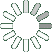Puzzle Command
Daily Puzzle
Number Logic #10
January 4, 2015Find a 6 digit number where:

The 1st digit is the sum of the 3rd, 5th, and 6th digit.
The 2nd digit is the number of 7s in the number.
The 3rd digit is the number of 0s in the number.
The 4th digit is equal to 7.
The 5th digit is equal to 6.
The 6th digit is even.

Every digit is lower than 8.

Puzzle Notes
6 digit numbers range from 100,000 to 999,999.
Enter in your solution below and click submit.